## Worldwide Governance Indicators and GDP per capita¶

Francisca Dias

In this analysis I want to see the relationship between 6 Worldwide Governance Indicators and their impact on GDP per capita.

• Is there a relationship between any of those indicators and GDP per capita?

• How strong is that relationship?

• What is the effect of each indicator in GDP per capita?

• Given any indicator, can GDP per capita be predicted?

## Steps I have taken to build the model¶

I want to know the impact of each one of these 6 Worldwide Governance Indicators separately (univariate model), and later include all of them in the model (multivariate). The sample includes all countries, and the indicators are from the year 2016. The dataset was taken from the World Bank.

I am applying two different python libraries for estimating the relationship between the dependent value (Log GDP per capita) and the independent variables (features) in the model: stats model and scikit learn. They will both lead to the same results.

I will interpret the results for both Univariate and Multivariate model, introduce some concepts and problems that may arise. I will approach multicollinearity and finally test/split the data so I can validate the model.

Data Preprocessing

• Clean the data: This includes renaming the columns, converting the values of all variables inot numeric, delete columns I will not need, map the name of features to acronymous, transpose the variables in order to have them as numpy arrays.

Simple Linear Regression

• Draw a heatmap, search for correlated variables, converting GDP per capita to log GDP per capita, plot variables, explain the logic behind linear regression, plot all countries in the map.

Univariate Regression with Stats Model

• Start with univariate model and deploy the statistics summary. Plot the OLS relationship between GE and GDP per capita.

Linear Regression with Stats Model

Linear Regression with Scikit-Learn

Multivariate Regression with Stats Model

• Now I include all variables in the model.

Multivariate Regression with Scikit-Learn

• Inlcude all variables within the model using sckikit-learn.

Model Optimization

## Data description¶

Dataset was taken from the World Bank Database.

Control of Corruption: Percentile Rank Control of Corruption captures perceptions of the extent to which public power is exercised for private gain, including both petty and grand forms of corruption, as well as "capture" of the state by elites and private interests. Percentile rank indicates the country's rank among all countries covered by the aggregate indicator, with 0 corresponding to lowest rank, and 100 to highest rank.

Government Effectiveness: Percentile Rank Government Effectiveness captures perceptions of the quality of public services, the quality of the civil service and the degree of its independence from political pressures, the quality of policy formulation and implementation, and the credibility of the government's commitment to such policies. Percentile rank indicates the country's rank among all countries covered by the aggregate indicator, with 0 corresponding to lowest rank, and 100 to highest rank.

Political Stability and Absence of Violence/Terrorism Political Stability and Absence of Violence/Terrorism measures perceptions of the likelihood of political instability and/or politically-motivated violence, including terrorism. Percentile rank indicates the country's rank among all countries covered by the aggregate indicator, with 0 corresponding to lowest rank, and 100 to highest rank.

Regulatory Quality: Percentile Rank Regulatory Quality captures perceptions of the ability of the government to formulate and implement sound policies and regulations that permit and promote private sector development. Percentile rank indicates the country's rank among all countries covered by the aggregate indicator, with 0 corresponding to lowest rank, and 100 to highest rank.

Rule of Law: Percentile Rank Rule of Law captures perceptions of the extent to which agents have confidence in and abide by the rules of society, and in particular the quality of contract enforcement, property rights, the police, and the courts, as well as the likelihood of crime and violence. Percentile rank indicates the country's rank among all countries covered by the aggregate indicator, with 0 corresponding to lowest rank, and 100 to highest rank.

Voice and Accountability: Percentile Rank Voice and Accountability captures perceptions of the extent to which a country's citizens are able to participate in selecting their government, as well as freedom of expression, freedom of association, and a free media. Percentile rank indicates the country's rank among all countries covered by the aggregate indicator, with 0 corresponding to lowest rank, and 100 to highest rank.

GDP per capita (constant 2010 US$): GDP per capita is gross domestic product divided by midyear population. GDP is the sum of gross value added by all resident producers in the economy plus any product taxes and minus any subsidies not included in the value of the products. It is calculated without making deductions for depreciation of fabricated assets or for depletion and degradation of natural resources. Data are in constant 2010 U.S. dollars. This dataset can be found here. ## Data Preprocessing¶ Import the libraries. In : import warnings warnings.filterwarnings("ignore") import pandas as pd import numpy as np  Import the dataset. In : gov_data = pd.read_csv('World_Bank_Governance_Data.csv')  Rename the columns. In : gov_data.rename(columns={'Country Name': 'Name', 'Country Code': 'Code', 'Series Name': 'Series', 'Series Code': 'Series_Code', '2016 [YR2016]': 'Values' }, inplace=True)  Convert the datatype from 'Object' to Numeric. In : gov_data = gov_data.convert_objects(convert_numeric=True)  Delete unnecessary columns. In : del gov_data['Name']  In : del gov_data['Series_Code']  Map the dataset to new features names. In : gov_data['Series'] = gov_data['Series'].map({'Control of Corruption: Percentile Rank': 'CC', 'Voice and Accountability: Percentile Rank':'VA', 'Government Effectiveness: Percentile Rank':'GE', 'Regulatory Quality: Percentile Rank':'RQ', 'Rule of Law: Percentile Rank':'RL', 'Political Stability and Absence of Violence/Terrorism: Percentile Rank':'PS'})  What are the features? • Control of Corruption: Percentile Rank • Voice and Accountability: Percentile Rank: • Government Effectiveness: Percentile Rank • Regulatory Quality: Percentile Rank • Rule of Law • Political Stability and Absence of Violence/Terrorism What is the response? • gdp per capita: Transform the dataset in order to have the features in columns so I can perform the analysis. In : gov_data_2 = gov_data.set_index('Code')  In : gov_data_3 = pd.pivot_table(gov_data_2,index=["Code"],values=["Values"],columns=["Series"], aggfunc=[np.sum])  In : gov_data_4 = pd.DataFrame(gov_data_3.to_records())  In : gov_data_4.columns.values = 'CC' gov_data_4.columns.values = 'GE' gov_data_4.columns.values = 'PS' gov_data_4.columns.values = 'RL' gov_data_4.columns.values = 'RQ' gov_data_4.columns.values = 'VA'  In : df1 = gov_data_4  Import the dataset related to GDP per capita. In : gdp_data = pd.read_csv('/Users/FranciscaDias/Kaggle_Temporary/***Kaggle_Competions***/8.Data_Extract_From_Global_Economic_Prospects/World_Bank_Governance/World_GDP_constant.csv')  In : gdp_data.head()  Out: Country Name Country Code Series Name Series Code 2016 [YR2016] 0 Afghanistan AFG GDP per capita (constant 2010 US$) NY.GDP.PCAP.KD 596.257638538373
1 Albania ALB GDP per capita (constant 2010 US$) NY.GDP.PCAP.KD 4711.98660680542 2 Algeria DZA GDP per capita (constant 2010 US$) NY.GDP.PCAP.KD 4846.41824654373
3 American Samoa ASM GDP per capita (constant 2010 US$) NY.GDP.PCAP.KD .. 4 Andorra AND GDP per capita (constant 2010 US$) NY.GDP.PCAP.KD ..

Rename the columns so I can merge with the first table.

In :
gdp_data.rename(columns={'Country Name': 'Name',
'Country Code': 'Code',
'Series Name': 'Series',
'Series Code': 'Series_Code',
'2016 [YR2016]': 'GDP_Values' }, inplace=True)

In :
del gdp_data['Name']

In :
del gdp_data['Series']

In :
del gdp_data['Series_Code']

In :
gdp_data = gdp_data.convert_objects(convert_numeric=True)

In :
gdp_data["log_gdp"] = np.log(gdp_data['GDP_Values'])

In :
complete = pd.merge(df1, gdp_data, on='Code', how='outer')

Out:
Code CC GE PS RL RQ VA GDP_Values log_gdp
0 ABW 88.942307 76.923080 94.285713 87.019234 88.942307 92.610840 NaN NaN
1 AFG 3.365385 9.615385 0.952381 3.846154 7.211538 21.182266 596.257639 6.390673
2 AGO 5.769231 13.461538 31.904762 13.461538 13.461538 16.748768 3606.644492 8.190533

## Simple Linear Regression¶

How can we measure the impact of governance indicators in GDP per Capita?

I will start by plotting a heatmap where I can see the correlation coefficient between variables.

Next I will show the difference between GDP per capita and the log of gdp per capita.

I will plot the linear relatinoship between Government Effectiveness (GE) and log gdp, first aggregate, and then separate so we can see the countries.

I will measure the relationship between GE and log gdp throught OLS and intepret the results.

In :
import seaborn as sns
import matplotlib.pyplot as plt
%matplotlib inline

sns.set(style="white")
# Compute the correlation matrix
corr = complete.corr()

f, ax = plt.subplots(figsize=(10, 7))
cmap = sns.diverging_palette(220, 10, as_cmap=True)

sns.heatmap(corr, annot=True, cmap=cmap, center=0,
square=True, linewidths=.5, cbar_kws={"shrink": .5});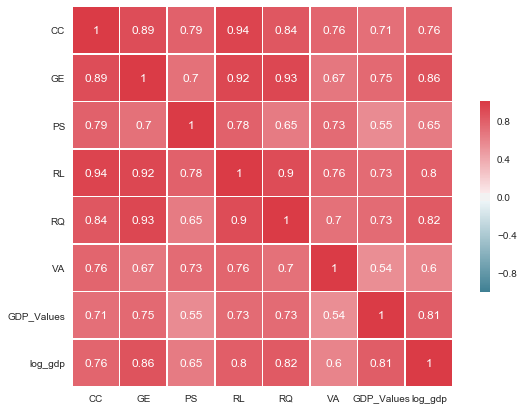As we would expect, most of Governance Indicators are positively correlated with gdp per capita. We can clearly see a redish square in the heatmap and it shows how significant the correlation is between these variables. This correlation is so strong that it can indicate a situation of multicollinearity. It is very likely that these variables, mainly CC, GE, PS, RL, RQ and VA give almost the same information, so multicollinearity really occurs.

In :
total = complete.isnull().sum().sort_values(ascending=False)
percent = (complete.isnull().sum()/complete.isnull().count()).sort_values(ascending=False)
missing_data = pd.concat([total, percent], axis=1, keys=['Total', 'Percent'])

Out:
Total Percent
log_gdp 44 0.198198
GDP_Values 44 0.198198
VA 21 0.094595
RQ 19 0.085586
RL 19 0.085586

We have to make sure there is no missing data.

In :
complete=complete.dropna(axis=0)


Below I am calculating correlation using two libraries: scipy and numpy

In :
from scipy.stats.stats import pearsonr
from numpy import corrcoef

a = complete['GE']
b = complete['log_gdp']
print(pearsonr(a,b))
print(np.corrcoef(a,b))

(0.85994932566577231, 2.7066652350392906e-53)
[[ 1.          0.85994933]
[ 0.85994933  1.        ]]


Our correlation estimation between target (log_gdp) and the GE indicator is 0.86 which is positive and strong.

This is also called partial correlation since we are trying to model the response (target) using just one predictor, in this case, GE.

In :
sns.distplot(complete['GDP_Values']);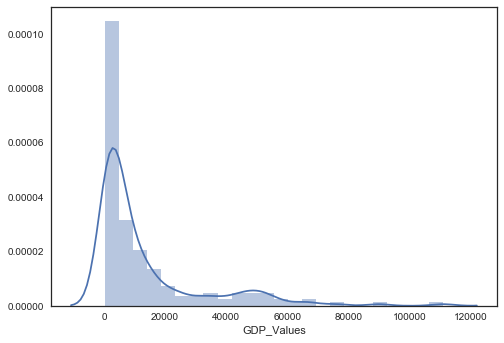mean larger than the median.

In :
sns.distplot(complete['log_gdp']);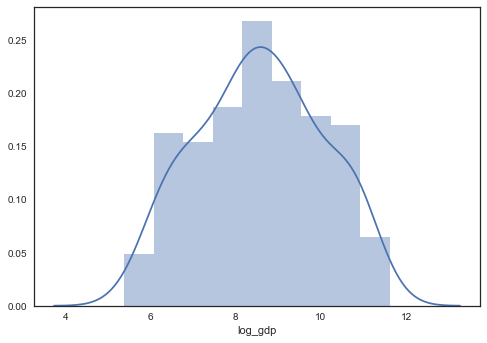In :
complete.GE.describe()

Out:
count    178.000000
mean      49.416595
std       28.031463
min        0.961538
25%       26.081731
50%       48.317308
75%       72.355768
max      100.000000
Name: GE, dtype: float64
In :
complete.plot(x='GE', y='log_gdp', kind='scatter')
plt.show()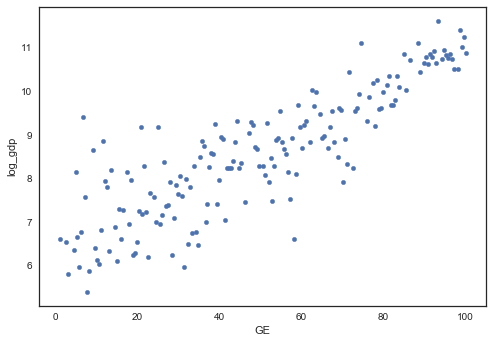This plot shows a positive relationship between GE and log GDP per capita.

Higher Government Effectiveness appears to be positively correlated with wealthier economies.

In order to describe this relationship I chooose the linear model, that can be translated to:

                               log gdp = β0 + β1GE + ui


• β0 is the intercept on the y axis

• β1 is the line´s slope

• ui, random error, are the deviations of observations from the line due to factors that were not considered in the model

Simple linear regression is an approach for predicting a quantitative response using a single feature (or "predictor" or "input variable"). It takes the following form:

y=β0+β1x

What does each term represent?

y is the response

x is the feature

β0 is the intercept

β1 is the coefficient for x

Together, β0 and β1 are called the model coefficients. To create your model, you must "learn" the values of these coefficients. And once we've learned these coefficients, we can use the model to predict log GDP!

In :
X = complete['GE']
y = complete['log_gdp']
f, ax = plt.subplots(figsize=(13, 9))
labels = complete['Code']
plt.scatter(X, y, marker='')
for i, label in enumerate(labels):
plt.annotate(label, (X.iloc[i], y.iloc[i]))
plt.xlabel('Government Effectiveness: Percentile Rank in 2016')
plt.ylabel('Log GDP (constant 2010 US$)') plt.title('OLS relationship between Government Effectiveness and Income') sns.regplot(x="GE", y="log_gdp", data=complete);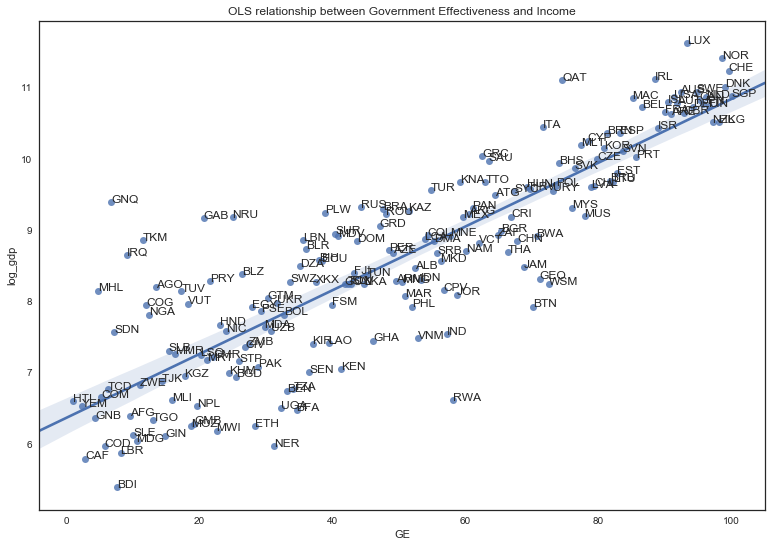By sorting the dataframe in descending order by log gdp values, if we call the head and tail methods we can see what are the countries with highest an lowest GPD, respectively. Countries with highest gdp per capita: • Luxembourg • Norway • Switzerland • Ireland • Qatar Countries with lowest gdp per capita: • Niger • Congo, Dem. Rep. • Liberia • Central African Republic • Burundi In : highest = complete.sort_values(['log_gdp'], ascending=[False]) highest.head(5)  Out: Code CC GE PS RL RQ VA GDP_Values log_gdp 111 LUX 97.596153 93.269234 97.619049 93.750000 93.75000 96.551727 111000.960314 11.617294 137 NOR 98.076920 98.557693 91.428574 99.519234 92.78846 100.000000 89818.322489 11.405544 33 CHE 96.153847 99.519234 95.714287 98.557693 98.07692 97.536949 75725.650669 11.234872 85 IRL 92.788460 88.461540 76.666664 90.384613 94.71154 93.596062 66787.139480 11.109266 154 QAT 79.807693 74.519234 76.190475 79.326920 74.03846 15.763547 66415.344142 11.103683 In : highest.tail(5)  Out: Code CC GE PS RL RQ VA GDP_Values log_gdp 133 NER 31.250000 31.250000 11.904762 29.807692 26.442308 34.482758 387.935612 5.960839 38 COD 7.692307 5.769231 4.285714 4.326923 7.692307 10.837439 387.444107 5.959572 104 LBR 26.442308 8.173077 25.714285 17.788462 15.865385 43.349754 352.646078 5.865465 31 CAF 9.134615 2.884615 7.142857 1.923077 5.769231 18.719212 325.720292 5.786039 13 BDI 10.576923 7.692307 5.238095 7.692307 20.673077 7.881773 218.283528 5.385795 To estimate the constant term β0, we need to add a column of 1’s to our dataset (consider the equation if β0 was replaced with β0x and xi=1) The X variable needs to be extended by a constant value(); the bias will be calculated accordingly. As we might remember, the formula of linear regression is y = bX + b In : complete.columns  Out: Index(['Code', 'CC', 'GE', 'PS', 'RL', 'RQ', 'VA', 'GDP_Values', 'log_gdp'], dtype='object') The steps to building and using a model are: • Define: This is where we choose the model. • Fit : Capture patterns from provided data. • Predict: • Evaluate: here we evauate model´s accuracy ## Linear Regression with Stats Model¶ #### Univariate Model¶ In : import statsmodels.api as sm  In : complete['const'] = 1 reg1 = sm.OLS(complete['log_gdp'],complete[['const', 'GE']]) results = reg1.fit() results.summary()  Out: Dep. Variable: R-squared: log_gdp 0.740 OLS 0.738 Least Squares 499.7 Thu, 16 Nov 2017 2.71e-53 14:25:49 -199.74 178 403.5 176 409.8 1 nonrobust coef std err t P>|t| [0.025 0.975] 6.3573 0.114 55.872 0.000 6.133 6.582 0.0448 0.002 22.353 0.000 0.041 0.049  Omnibus: Durbin-Watson: 6.624 1.977 0.036 9.779 0.159 0.00753 4.103 115 In : import statsmodels.formula.api as smf  In : reg2 = smf.ols(formula = 'log_gdp ~ GE', data = complete) results = reg2.fit() results.summary()  Out: Dep. Variable: R-squared: log_gdp 0.740 OLS 0.738 Least Squares 499.7 Thu, 16 Nov 2017 2.71e-53 14:25:49 -199.74 178 403.5 176 409.8 1 nonrobust coef std err t P>|t| [0.025 0.975] 6.3573 0.114 55.872 0.000 6.133 6.582 0.0448 0.002 22.353 0.000 0.041 0.049  Omnibus: Durbin-Watson: 6.624 1.977 0.036 9.779 0.159 0.00753 4.103 115 We can now write our estimated relationship as log gdp = 0.04 GE + 6.357 This equation describes the line that best fits our data. Let´s calculate the average GE in our dataset: In : complete.GE.mean()  Out: 49.41659460509761 Let us estimated the expected log of gdp per capita with an average GE of 49: In : y = 0.04 * 49 + 6.357 y  Out: 8.317 Just a reminder that the beta of each feature becomes its unit change measure, which corresponds to the change the outcome will have if the feature increases one unit. For instance, let us see what happens to log_gdp if we increase one point on GE, from 49 to 50: In : y = 0.04 * 50 + 6.357 y  Out: 8.357 In : y0=8.317 y1=8.357 (y1-y0)*100  Out: 3.9999999999999147 This result can be interpreted as the following: An increase of unit change in GE, leads to an increase in log GDP by 4%. This is the same as calling the predict() method: If we hit the command results.predict() we will get an array of all predicted log gdp for every value of GE. We can compare the observed and predicted values of log GDP by plotting them on the graph below. In : f, ax = plt.subplots(figsize=(12, 9)) sns.regplot(x="GE", y = results.predict(), data = complete, label='predicted') sns.regplot(x="GE", y = complete['log_gdp'], data = complete,label='observed') plt.xlabel('Government Effectiveness: Percentile Rank in 2016') plt.ylabel('Log GDP (constant 2010 US$)')
plt.title('OLS relationship between Government Effectiveness and Income')
plt.legend();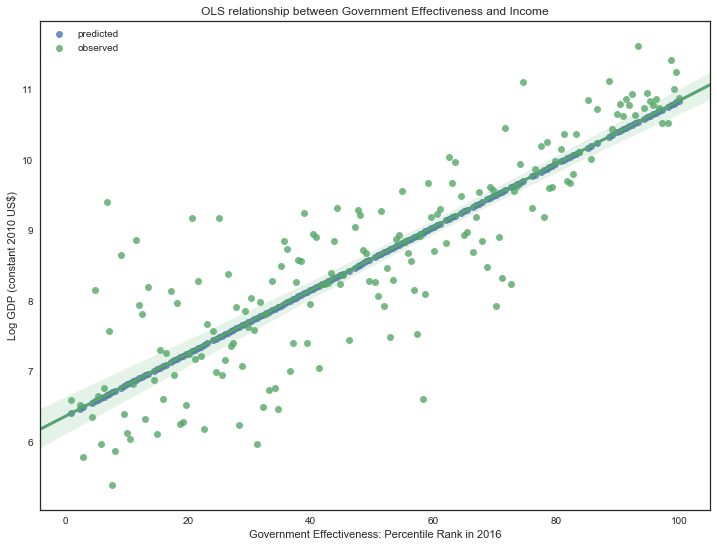## Linear Regression with Scikit-Learn¶

In :
from sklearn import linear_model

In :
linear_regression = linear_model.LinearRegression()
linear_regression

Out:
LinearRegression(copy_X=True, fit_intercept=True, n_jobs=1, normalize=False)
In :
complete.head()

Out:
Code CC GE PS RL RQ VA GDP_Values log_gdp const
1 AFG 3.365385 9.615385 0.952381 3.846154 7.211538 21.182266 596.257639 6.390673 1
2 AGO 5.769231 13.461538 31.904762 13.461538 13.461538 16.748768 3606.644492 8.190533 1
3 ALB 41.346153 52.403847 55.238094 39.423077 60.576923 51.724136 4711.986607 8.457865 1
5 ARE 88.461540 90.865387 60.952381 79.807693 80.288460 19.211823 40864.249847 10.618011 1
6 ARG 46.153847 60.576923 53.809525 39.903847 33.653847 65.517242 10148.510147 9.225082 1
In :
feature_cols = ['GE', 'const']
X = complete[feature_cols]
y = complete.log_gdp

In :
linear_regression.fit(X, y)

Out:
LinearRegression(copy_X=True, fit_intercept=True, n_jobs=1, normalize=False)
In :
# print intercept and coefficients
print(linear_regression.intercept_)
print(linear_regression.coef_)

6.35730082863
[ 0.04479802  0.        ]


## Multivariate Regression Model with Stats Model¶

We can add more variables to our model. So far we only focused on the impact of GE on log gdp, but we still have other variables that we can include in the model. For this purpose we go from a bivariate model to a multivariate model that reflects the additional variables.

In :
X1 = ['const', 'GE', 'CC', 'PS', 'RL', 'RQ', 'VA']

In :
# Estimate an OLS regression for all set of variables
reg3 = sm.OLS(complete['log_gdp'], complete[X1])
results = reg3.fit()
results.summary()

Out:
Dep. Variable: R-squared: log_gdp 0.761 OLS 0.753 Least Squares 90.81 Thu, 16 Nov 2017 1.50e-50 14:25:50 -192.03 178 398.1 171 420.3 6 nonrobust
coef std err t P>|t| [0.025 0.975] 6.1774 0.125 49.536 0.000 5.931 6.424 0.0364 0.006 5.601 0.000 0.024 0.049 -0.0070 0.006 -1.152 0.251 -0.019 0.005 0.0107 0.003 3.249 0.001 0.004 0.017 -0.0025 0.007 -0.335 0.738 -0.017 0.012 0.0107 0.006 1.879 0.062 -0.001 0.022 0.0002 0.003 0.055 0.957 -0.006 0.007
 Omnibus: Durbin-Watson: 5.674 1.881 0.059 6.159 0.267 0.046 3.738 312
In :
reg4 = smf.ols(formula = 'log_gdp ~ GE + CC + PS + RL + RQ + VA', data = complete)
results = reg4.fit()
results.summary()

Out:
Dep. Variable: R-squared: log_gdp 0.761 OLS 0.753 Least Squares 90.81 Thu, 16 Nov 2017 1.50e-50 14:25:50 -192.03 178 398.1 171 420.3 6 nonrobust
coef std err t P>|t| [0.025 0.975] 6.1774 0.125 49.536 0.000 5.931 6.424 0.0364 0.006 5.601 0.000 0.024 0.049 -0.0070 0.006 -1.152 0.251 -0.019 0.005 0.0107 0.003 3.249 0.001 0.004 0.017 -0.0025 0.007 -0.335 0.738 -0.017 0.012 0.0107 0.006 1.879 0.062 -0.001 0.022 0.0002 0.003 0.055 0.957 -0.006 0.007
 Omnibus: Durbin-Watson: 5.674 1.881 0.059 6.159 0.267 0.046 3.738 312

Notes to the Results:

• The R squared increased when we add more variables to our model, as we would expect.
• For this reason we should look at the adjusted R squared since this considers the complexity of the model and give us a more realistic measure.
• One good tip when considering using adjusted is to make the ratio between R squared and adjusted R squared; If exceeds 20%, it means that we probably add variables to our model that are redundant.
• In our case this ratio is 1% over the R square.
• We should also be cautious with p-values. Low p-values (using p < 0.05 as a rejection rule) implies that the effect of these features on log gdp is statistically significant. Therefore the use of CC, RL, RQ and VA can challenge our model.
• When it comes to Cond. No, when the score is greather than 30, such is our case, it signals unstable numerical results. This unstabiity is due to multicollinearity.

Remember the correlation matrix from the beginning?

In :
correlation_matrix = complete.iloc[:, 1:7]
corr = correlation_matrix.corr()
corr

Out:
CC GE PS RL RQ VA
CC 1.000000 0.880305 0.756812 0.938637 0.832236 0.759427
GE 0.880305 1.000000 0.649924 0.914203 0.927020 0.649148
PS 0.756812 0.649924 1.000000 0.753687 0.606017 0.707947
RL 0.938637 0.914203 0.753687 1.000000 0.889127 0.754628
RQ 0.832236 0.927020 0.606017 0.889127 1.000000 0.678350
VA 0.759427 0.649148 0.707947 0.754628 0.678350 1.000000

We can see that there's strong correlation between variables, they are all above 0.5.

Another way to see associations among variables is to use the eigenvectors. They recombine the variance among the variables, therefore it is easier to spot the multicollinearity.

In :
# Consider the all columns except code, Value GDP and log gdp
variables = complete.columns[1:-3]
variables

Out:
Index(['CC', 'GE', 'PS', 'RL', 'RQ', 'VA'], dtype='object')
In :
eigenvalues, eigenvectors = np.linalg.eig(corr)
eigenvalues

Out:
array([ 4.91809627,  0.53362901,  0.29883127,  0.13988831,  0.04983594,
0.0597192 ])
In :
eigenvectors

Out:
array([[ 0.43069114,  0.02823625, -0.08456242, -0.70656827,  0.55409421,
-0.0172613 ],
[ 0.4197663 ,  0.41183626, -0.11222426,  0.12076426, -0.18642339,
0.76958117],
[ 0.36860607, -0.62183383, -0.60917155,  0.32031261,  0.06089178,
0.00736871],
[ 0.43784084,  0.11877655, -0.07649235, -0.27189413, -0.7186297 ,
-0.44495099],
[ 0.41214905,  0.43040891,  0.13166396,  0.55172106,  0.36784208,
-0.43340744],
[ 0.37531256, -0.49351453,  0.7654923 ,  0.07248837, -0.05274168,
0.14686447]])

Let's investigate the eigenvector on last column, index 5:

In :
eigenvectors[:, 5]

Out:
array([-0.0172613 ,  0.76958117,  0.00736871, -0.44495099, -0.43340744,
0.14686447])
In :
print(variables, variables)

GE RL


Removing these two columns would be the best solution.

Now we want our model to generalize well on new data. Therefore we need to test it in that situation.

## Model Optimization¶

In :
feature_cols = ['CC', 'PS','RQ', 'VA']
X = complete[feature_cols]
y = complete.log_gdp


Models' practical value come from making predictions on new data, so we should measure performance on data that wasn't used to build the model. Therefore we should split the data and test the model accuracy on data it hasn't seen before - validation data.

In :
from sklearn.model_selection import train_test_split

In :
train_X, val_X, train_y, val_y = train_test_split(X, y,random_state = 0)

In :
from sklearn import linear_model
linear_regression = linear_model.LinearRegression()
linear_regression.fit(train_X, train_y)

Out:
LinearRegression(copy_X=True, fit_intercept=True, n_jobs=1, normalize=False)
In :
# get predicted prices on validation data
val_predictions = linear_regression.predict(val_X)

In :
from sklearn.metrics import mean_absolute_error
print(mean_absolute_error(val_y, val_predictions))

0.689606143226

In [ ]: